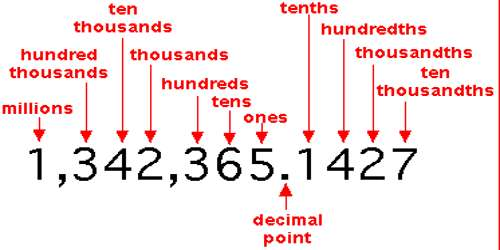Decimals of Thousandths

When we write numbers with thousandths using decimals we use a decimal point and places to the right of this decimal point. The thousandth place is three places to the right of the decimal point.

A decimal is a fractional number and is indicated by digits after a period which is called a decimal point. Tenths have one digit after the decimal point. The decimal 0.8 is pronounced “eight tenths” or “zero point eight”. It is equal to the fraction 8/10.

Hundredths have two digits after the decimal point. The decimal 0.36 is pronounced “thirty-six hundredths” or “zero point thirty-six”. It is equal to the fraction 36/100.

Thousandths, as the word implies, is the place value that represents one thousandth of a whole. It is also one tenth of a hundredth and one hundredth of a tenth. Thousandths follow a similar pattern. They have three digits after the decimal point. The decimal 0.749 is pronounced “seven hundred forty-nine thousandths” or “zero point seven forty-nine”.

There may be zeros after the decimal point. The decimal 0.064 is pronounced “sixty-four thousandths” or “zero point zero sixty-four”.

There may be zeros after the decimal point. The decimal 0.064 is pronounced “sixty-four thousandths” or “zero point zero sixty-four”. A decimal number may be larger than 1. The word and may be used to indicate the decimal point so it should not be used in other parts of the name of the decimal. The decimal 234.987 could be pronounced Two hundred thirty-four AND nine hundred eighty-seven thousandths.Decimals – Ten-Thousandths

Thousandths follow a similar pattern. They have three digits after the decimal point. The decimal 0.749 is pronounced “seven hundred forty-nine thousandths” or “zero point seven four nine”.

Ten-thousandths have four digits after the decimal point.

There may be zeros after the decimal point. The decimal 0.064 is pronounced “sixty-four thousandths” or “zero point zero six four”.

One decimal place to the left of the decimal point is the ones place. One decimal place to the right of the decimal place is the tenths place.

Information Sources: